# Plot the decision boundaries of a VotingClassifier¶

Plot the decision boundaries of a VotingClassifier for two features of the Iris dataset.

Plot the class probabilities of the first sample in a toy dataset predicted by three different classifiers and averaged by the VotingClassifier.

First, three exemplary classifiers are initialized (DecisionTreeClassifier, KNeighborsClassifier, and SVC) and used to initialize a soft-voting VotingClassifier with weights [2, 1, 2], which means that the predicted probabilities of the DecisionTreeClassifier and SVC each count 2 times as much as the weights of the KNeighborsClassifier classifier when the averaged probability is calculated.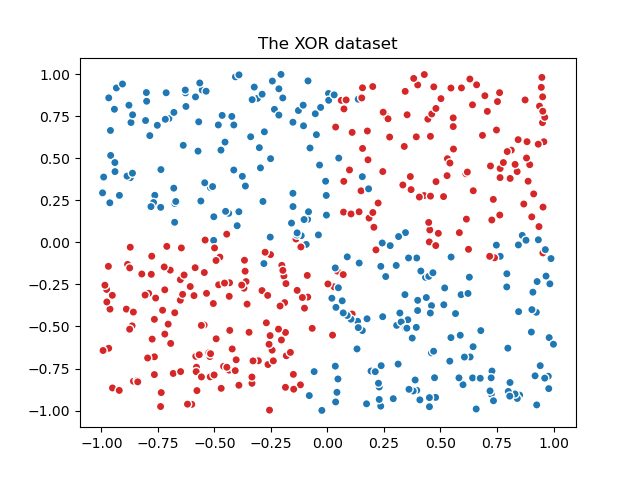from itertools import product

import matplotlib.pyplot as plt

from sklearn import datasets
from sklearn.ensemble import VotingClassifier
from sklearn.inspection import DecisionBoundaryDisplay
from sklearn.neighbors import KNeighborsClassifier
from sklearn.svm import SVC
from sklearn.tree import DecisionTreeClassifier

X = iris.data[:, [0, 2]]
y = iris.target

# Training classifiers
clf1 = DecisionTreeClassifier(max_depth=4)
clf2 = KNeighborsClassifier(n_neighbors=7)
clf3 = SVC(gamma=0.1, kernel="rbf", probability=True)
eclf = VotingClassifier(
estimators=[("dt", clf1), ("knn", clf2), ("svc", clf3)],
voting="soft",
weights=[2, 1, 2],
)

clf1.fit(X, y)
clf2.fit(X, y)
clf3.fit(X, y)
eclf.fit(X, y)

# Plotting decision regions
f, axarr = plt.subplots(2, 2, sharex="col", sharey="row", figsize=(10, 8))
for idx, clf, tt in zip(
product([0, 1], [0, 1]),
[clf1, clf2, clf3, eclf],
["Decision Tree (depth=4)", "KNN (k=7)", "Kernel SVM", "Soft Voting"],
):
DecisionBoundaryDisplay.from_estimator(
clf, X, alpha=0.4, ax=axarr[idx, idx], response_method="predict"
)
axarr[idx, idx].scatter(X[:, 0], X[:, 1], c=y, s=20, edgecolor="k")
axarr[idx, idx].set_title(tt)

plt.show()


Total running time of the script: (0 minutes 0.943 seconds)

Related examplesPlot class probabilities calculated by the VotingClassifier

Plot class probabilities calculated by the VotingClassifier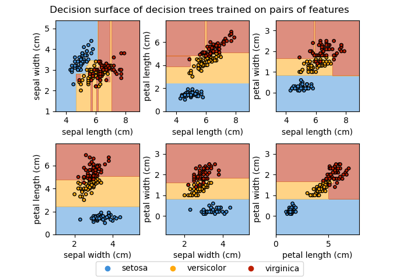Plot the decision surface of decision trees trained on the iris dataset

Plot the decision surface of decision trees trained on the iris dataset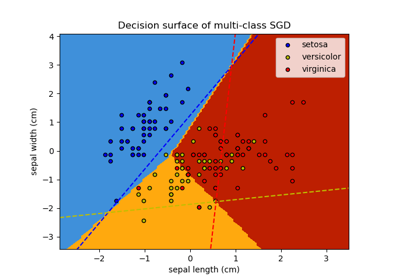Plot multi-class SGD on the iris dataset

Plot multi-class SGD on the iris dataset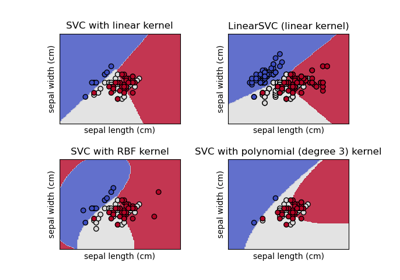Plot different SVM classifiers in the iris dataset

Plot different SVM classifiers in the iris dataset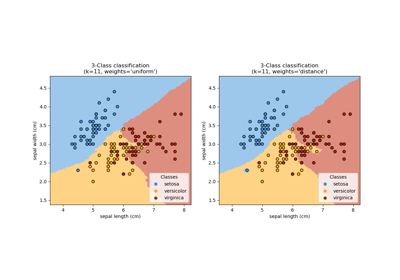Nearest Neighbors Classification

Nearest Neighbors Classification

Gallery generated by Sphinx-Gallery